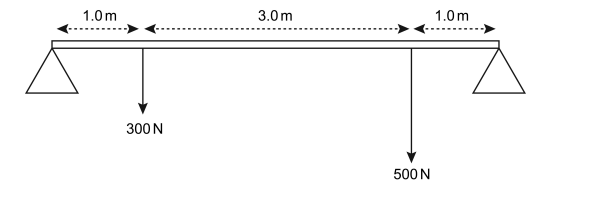# IMAT 2016 Q58 [Upwards Forces]

The diagram shows a uniform horizontal beam of negligible mass, 5.0 m long, placed on two supports, one at each end. It has a 300 N weight placed 1.0 m from one end and a 500 N weight placed 1.0 m from the other end. Both weights act vertically on the beam as shown in the diagram.What are the upward forces from the two supports acting on the beam?

A. 340 N and 460 N
B. 300 N and 500 N
C. 240 N and 560 N
D. 400 N and 400 N
E. 360 N and 540 N